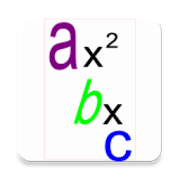# Solve Quadratic Equation with Steps - Lumos Educational App Store51
Price -FREE
\$0

#### DESCRIPTION:

Solve Quadratic Equation is an app used in the education sector to solve quadratic mathematical equation. This app solve the equation and also show the steps it uses to solve the mathematical equation be it completing the square method or the quadratic formulae method. Try it out and contact me if you love it and wants something like that for your projects, app development, mathematics subject, solve algebrae problems

#### OVERVIEW:

Solve Quadratic Equation with Steps is a free educational mobile app By .It helps students in grades HS practice the following standards HSN.CN.C.7.

This page not only allows students and teachers download Solve Quadratic Equation with Steps but also find engaging Sample Questions, Videos, Pins, Worksheets, Books related to the following topics.

1. HSN.CN.C.7 : Solve quadratic equations with real coefficients that have complex solutions..

HS

#### STANDARDS:

HSN.CN.C.7

Developer:

Software Version: 4.1.5

Category: Education

### RELATED APPSEdSearch WebSearch